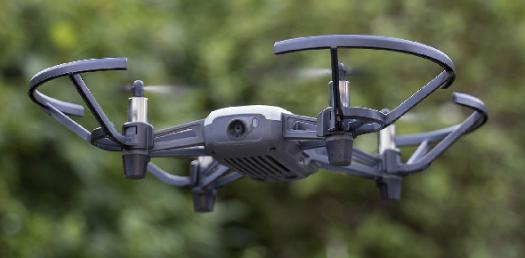# Air And Space Vocabulary Test

5 QuestionsSettingsThis test is vocabulary only. It covers the information found in the Air and Space Technology chapter.

Related Topics
• 1.
________________ is the study of how things fly.
• A.

Aerodynamics

• B.

S

• C.

S

• D.

S

• E.

S

• 2.
________________ is the force that pulls objects toward the center of the earth.
• A.

Gravity

• B.

S

• C.

S

• D.

S

• E.

Xz

• 3.
_________________ can be described as an object in motion resisting any change in its speed and direction unless another force acts on it.
• A.

Inertia

• B.

S

• C.

S

• D.

S

• E.

S

• 4.
_________________ is the connection between how fast an object isa moving and the mass of the object.
• A.

S

• B.

S

• C.

S

• D.

Momentum

• E.

S

• 5.
The force of air on a mvoing object is called fluid friction or _________.
• A.

Drag

• B.

S

• C.

S

• D.

S

• E.

S